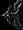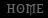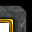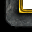# Mathematics - Formulas

Tutorial By Chocobo

I will post there some formulas used by Warcraft 3 to calculate dummy values, like Evasion with Effective Hp, Critical Strike with Effective Damage, blabla.. and also an added thing for Experience calculations.

1.1 Evasion Myths

There are a lot of myths for Evasion, including those :

A unit with 50% evasion has 50% more hp.

Totally wrong. If 50% evasion does 50% more hp, 100% will do :

A unit with 100% evasion has 100% more hp.

Won't it?

Also, there is a Myth that Evasion isn't stackable. It is since you can try to add as many evasion you want, they will all stack. But 2x 15% evasion isn't 30% evasion, the formula X+Y=Z doens't work.

Remember with Evasion : A missed attack that deal splashes stills dealing damage. It deals 50% of normal damages.

1.2 Calculating Effective Hp for Evasion

The formula you can use to calculate the effective hp for evasion is :

a = b*(100/(100-c))*(1+(d*e))

a = Effective Hp
b = Base Hp
c = Evasion Percentage
d = Armor
e = Armor Multiplier

But, wait. You do not know yet what is Effective Hp. It is the total damage a unit can handle before dying, excluding hp regeneration, excluding Spells, and damage modifiers (example : piercing vs divine, magic vs heavy...).

If you are using a hero to calculate the formula, it will do that formula :

a = (b*c)(100/(100-d))*(1+((e*f)*g))

a = Effective Hp
b = Strength of Hero
c = Hp given per Strength Point
d = Evasion Percentage
e = Agility
f = Armor per Agility point
g = Armor Multiplier

1.3 Effective Hp vs Base Hp

You can think that Effective Hp is better than Base Hp, but it can be sometimes wrong.

Effective Hp Demon Hunter Lv 1 = 830 Hp
Base Hp Demon Hunter Lv 1 = 575 Hp

We will divide Effective Hp by Base Hp, and it will return a decimal value :

c = a/b ~ 1.4438
a = 830
b = 575
c = Spell Multiplier Dealt

With that "c", every spell that is focused on the hero will take :

e = d*c

c = Spell Multiplier Dealt
d = Damage Dealt of the Spell
e = Total Damage

So, the demon hunter with level 1 evasion will take (Damage*1.4438) damage from abilities. So does healing abilities.

Because that new value we called "c", the hero is less powerful against abilities (but if we do not learn evasion it still with only 575 hp without any multiplier), but can fight better against weapon battles (melee or ranged battle without any spell). Also, Heal and Holy Light powers are increased.

g = f*c

c = Spell Multiplier Dealt
f = Healed Amount

-> g = 25*1.4438 ~ 36.095 hp (heal)

-> g = 200*1.4438 ~ 288.76 hp (holy light)

g=f*c works only for weapon battles.

From Whiteshock : Evasion doesn't raise hp because it just absorbs the damage. 200 dmg on a unit with 190 hp, zero armor, and 50% evasion will still kill it if it doesn't miss.

Comment : Yes, it absorbs, but it doesn't really absorb damages since splash attacks deal only (and ever if evasion runs) 50% damage when you evade.

1.4 Stacking Evasion

Evasion is stackable, formulas can be found in these links :
http://www.thehelper.net/forums/showpost.php?p=380544&postcount=9
http://www.thehelper.net/forums/showpost.php?p=376530&postcount=5
http://www.thehelper.net/forums/showpost.php?p=376865&postcount=6

2.1 Critical Strike Myths

There are a lot of myths for Critical Strike, including those :

When a blademaster learns critical strike to level 1 he deals 2/0.15 (~13.33333....) more damage.

When a blademaster acquires a Searing Blade he can't use the item critical strike.

The second myth is totally wrong, and there can be multiple critical strikes at same time, dealing a powerful hit.

We will see the first myth in some minutes.

2.2 Calculating Damage per Second

The formula used to calculate Dmg/s is :

a = ((b+c)/2)/d

a = Dmg/s
b = Minimal Damage
c = Maximal Damage
d = Attack Cooldown

This formula is easy to understand, it calculates the average of min~max damage, then divide it by cooldown.

2.3 Calculative Effective Attack Speed

The formula used to calculate the Effective Attack Speed is :

a = b*(100/(100+c))

a = Effective Attack Speed
b = Base Attack Cooldown
c = Bonuses

Bonuses are all values mixed in a number, which is equal to the sum of attack speed bonuses.

To calculate the Effective Attack Speed of a hero, you should use this formula :

a = b*(100/(100+c+(d*(e*100))))

a = Effective Attack Speed
b = Base Attack Cooldown
c = Bonuses
d = Agility
e = Attack Speed Bonus per Agility Point

Bonuses get less valuable as you get more it. Because that, you may want to boost an another effective value.

2.4 Calculating Damage/s or Damage/Attack with Critical Strike

To calculate the Damage per Second with Critical Stirke, this formula will do it :

a = (b*(100/(100+c+(d*(e*100)))))*(((f*g-1)/100)+1)

a = Damage per Second
b = Base Attack Cooldown
c = Bonuses
d = Agility
e = Attack Speed Bonus per Agility Point
f = Critical Percentage
g = Critical Multiplier

If you want to calculate the Damage per Attack with Critical Strike, you will go on this :

a = (((b+c)/2)/d)*(((e*f-1)/100)+1)

a = Dmg/s
b = Minimal Damage
c = Maximal Damage
d = Attack Cooldown
e = Critical Percentage
f = Critical Multiplier

2.5 Calculating Damage on a Double Critical Strike

You can simply use this formula to calculate that :

a = (b*c*d...)

a = Total Multiplier
b = First Multiplier
c = Second Multiplier
d = Third Multiplier
...

Example : We have 4 critical strikes that deals respectivly 1.6x (b), 2.1x (c), 3.6x (d), and 2.6x (e).
Leading us to : a = (1.6*2.1*3.6*2.6) = 31.4496x
We can also calculate for each Critical Strike.
a = (b*c) = 1.6*2.1 = 3.36x
a = (b*d) = 1.6*3.6 = 5.76x
a = (b*e) = 1.6*2.6 = 4.16x
a = (c*d) = 2.1*3.6 = 7.56x
a = (c*e) = 2.1*2.6 = 5.46x
a = (d*e) = 3.6*2.6 = 9.36x
a = (b*c*d) = 1.6*2.1*3.6 = 12.096x
a = (b*c*e) = 1.6*2.1*2.6 = 8.736x
a = (b*d*e) = 1.6*3.6*2.6 = 14.976x
a = (c*d*e) = 2.1*3.6*2.6 = 19.656x

2.6 Calculating the percentage for a Double Critical Strike

The formula used to calculate a Double Critical Strike is :

a = (b*c*d...)

a = Percentage to perform the Double Critical Strike
b = First Percentage
c = Second Percentage
d = Third Percentage
....

Like Calculating damage on a Double Critical Strike.

Example : We have 3 critical strikes of 15% each, which leads us to :
a = (0.15*0.15*0.15) = 0.003375 = 0,3375%
And also.. : a = (0.15*0.15) = 0.0225 = 2.225% (2 criticals)

3.1 Bouncing Attack Myths

In Battle.Net, only a small amount of people research the Upgrade Moon Glaive, whereas a lot of people like learning Chain Lightning with the Far Seer.

Why? We will explain it in some seconds.

3.2 Calculating X's Bounce Damage

The formula used to calculate the damage dealt at a fixed bounce of your wants, you will go on this :

a = b * (c/100) ^ (d)

a = Damage Dealt at bounce d
b = Base Damage of the ability
c = Damage Reduction of the ability
d = Fixed bounce number you wanted

Example : A huntress attacks multiple units, dealing 17 damage to first target and 50% damage is reduced per bounce. We will find the damage at the second bounce.

We use the formula a=b(c/100)^d, a=17(50/100)^2 = 17(0.5)^2 = 17(0.25) = 4.25

The second bounce of the huntress deals only 4.25 damage to the third unit that is hurted by the moon glaive.

Whereas with Chain Lightning level 3 at the lastest bounce deals :

a=180(85/100)^7 = 180(0.85)^7 = 180(0.3205) ~ 57.703859

Chain Lightning deals at the 8st target around 57.703859 damage, which punishes easily a worker for only 120 mana.

4. Damage Dices

Damage Dices are used to generate a random value to extend the damage power. It is wrote like this formula : xdy

x = Amount of Dices
y = Sides per Dice

And, it is read as "x y-sided dice".

To calculate the minimal and maximal damage of a base damage, we will use this formula :

a = c + d
b = c + (d*e)

a = Minimal Damage
b = Maximal Damage
c = Base Damage
d = Number of Dices
e = Sides per Dice

4.1 Plan of Hero Making (Section - Map makers only)

Warcraft III has some RPG aspects, like saving a hero in a campaign, or using the dice damage system. Do not read what is after this, unless you are going to make a map that is based on Warcraft III Melee System.

4.2 Units (Section - Normal Units)

Basically, most of high leveled creeps (Dragons, Ursa Warriors...) gets high damage and a lot of hit points.

The most known damage for mercernaries is "18d3", meaning :

(a+18)
(a+54)

"a" meaning Base Damage, (a+18) equal to minimal damage, (a+54) equal to maximal damage.

Example, 38-70 damages. The "d3" part means that the roll can only have 3 possibilities, equal to 18*roll. So, that unit with 38-70 damage can do only 38, 54, or 70 damages.

For normal units :
- You will go on 2d4 or 4d2 on light units.
- You will go on 2d2 or 1d4 on magic attack units.
- You will go on 3d6, 6d2, 6d3, 6d4 or 4d4 for heavy units.
- You will go on 5d4, 4d5, 3d7, 7d4 or 6d5 for siege units.
- You will go on 3d3, 2d5, 5d2, 5d3 or 2d6 for other units.

For upgrades, you will go on planning one extra dice every level, unless you want the upgrade to be ultra-strong or ultra-weak.

4.3 Units (Section - Heroes)

All heroes has got that dice type (depends if melee or ranged) depending of their stats base :

Strength : 2d6
Agility : 2d12
Intelligence : 2d4

For agility heroes, agility isn't really the biggest one because of the 2d12 allowing them to do a max of +24 damage, it is why their support isn't really required.

Also, most of heroes have got that movement speed :

Average : 270 (tanker heroes)
Above Average : 300 (some ranged heroes)
Fast : 320 (most of heroes)

Any heroes that haven't got a really "super" ability (exemple : Flame Strike) gets a lower cooldown instead of the 2.13 base (maybe it goes lower than that).

Most of non-agility heroes starts with 2 base armor, instead of 0 for agility heroes.

Any heroes start about 50 to 60 stats points, starting each with a base of 5. That means that 5 str is counted as 0 stat point, below 0, it counts as a negative stat point. Also, each hero gets exactly 6.00 stats points per level, Lich is only an exception because of Frost Nova with Dark Ritual for Mana. You must balance as much possible the stats for a heroes, so it doesn't get killed too fast, having enough mana to cast at least 2-3 times an ability, and having enough armor/attack speed.

About stats, Intelligence isn't really good at ending, because you will end with a lot of mana you can't wash in a battle (exception : Sea Naga Witch for the overpowered Mana Shield), it offers also no survival chances in battle since it only applies to mana, unless you have Mana Shield (that ignores armor, do not forget it). You may go on Strength of Agility's linear exponential overgrowth, but Strength still the best. It beats Agility at start, and also Agility gets out after a long time. Strength protects also from magics while agility doesn't, agility heroes will require a lot of periaps of vitality to survive in a battle, especially if they are melee. But notice Intelligence heroes are highly prized because they have got a huge pool of mana (especially Lich with Dark Ritual for Mana), while Agility Heroes are only going on a FFA start (quite useless since you can revive a level 1 hero at the lowest price possible ever).

4.4 Abilities (Section - Heroes)

Normal Spells shouldn't get a huge cooldown or a huge mana cost, because ultimates are for that. You must be able to cast at least 2 or 3 times an ability at level 1 with a level 1 hero, or that hero will become very useless in game. You must fit the good skills for each hero, depending on their base stats, but also depending on the hero's skin, like Lich casts Storm Bolt on a Mountain King? Never give the Bash ability to agility heroes, because they will go in a ultra frenzy, exemple, give Bash to a Blademaster and you'll see the result.

Most of hero skills goes on that way :
Strength : Active Offense, Active Offense, Passive/Aura, Ultimate Summon
Agility : Active Offense, Active Utility, Active Single Target/Passive, Ultimate AoE
Intelligence : Active Offense, Summon, Passive/Aura, Ultimate Summon/Utility

If your stunning ability is going to do more damage than Storm Bolt, stunning more time than Entanging Roots, doing more area of effect than Flame Strike, or healing more life than Holy Light, you are going to do something wrong, and you might want rethink.

5. Experience

Warcraft III Heroes are basically based on experience for their levels.

5.1 Formula Used?

A formula is used to calculate the experience required. At N level, it requires it's previous experience required at N-1 level.

a = Previous Value Factor
b = Level Factor
c = Constant Factor
XP for level N = ((XP for level N - 1) * a) + (N * b) + c

or :

ƒ(N)=((f(N-1)-1)*a)+(N*b)+c

Example for basic values for melee games :

a = 1.00
b = 100
c = 100

Level 1 = 0
Level 2 = ((Level (1-0) Exp)*1)+(1*100)+100 = 0+100+100 = 200
Level 3 = ((Level (2-1) Exp)*1)+(2*100)+100 = 200+200+100 = 500
Level 4 = ((Level (3-1) Exp)*1)+(3*100)+100 = 500+300+100 = 900
Level 5 = ((Level (4-1) Exp)*1)+(4*100)+100 = 900+400+100 = 1400
Level 6 = ((Level (5-1) Exp)*1)+(5*100)+100 = 1400+500+100 = 2000
Level 7 = ((Level (6-1) Exp)*1)+(6*100)+100 = 2000+600+100 = 2700
Level 8 = ((Level (7-1) Exp)*1)+(7*100)+100 = 2700+700+100 = 3500
Level 9 = ((Level (8-1) Exp)*1)+(8*100)+100 = 3500+800+100 = 4400
Level 10 = ((Level (9-1) Exp)*1)+(2*100)+100 = 4400+900+100 = 5400

5.2 Other Formulas?

Acehart (1)

Acehart (2) - Works only with the number 5

Acehart (3) - Explanation of f(x) = A*f(x-1) + B*x + C

Acehart (4) - Explanation of XN = A^N * X0 + B * sum(i = 1, n, A^(N - i) * i) + C * (A^N - 1) / (A - 1)